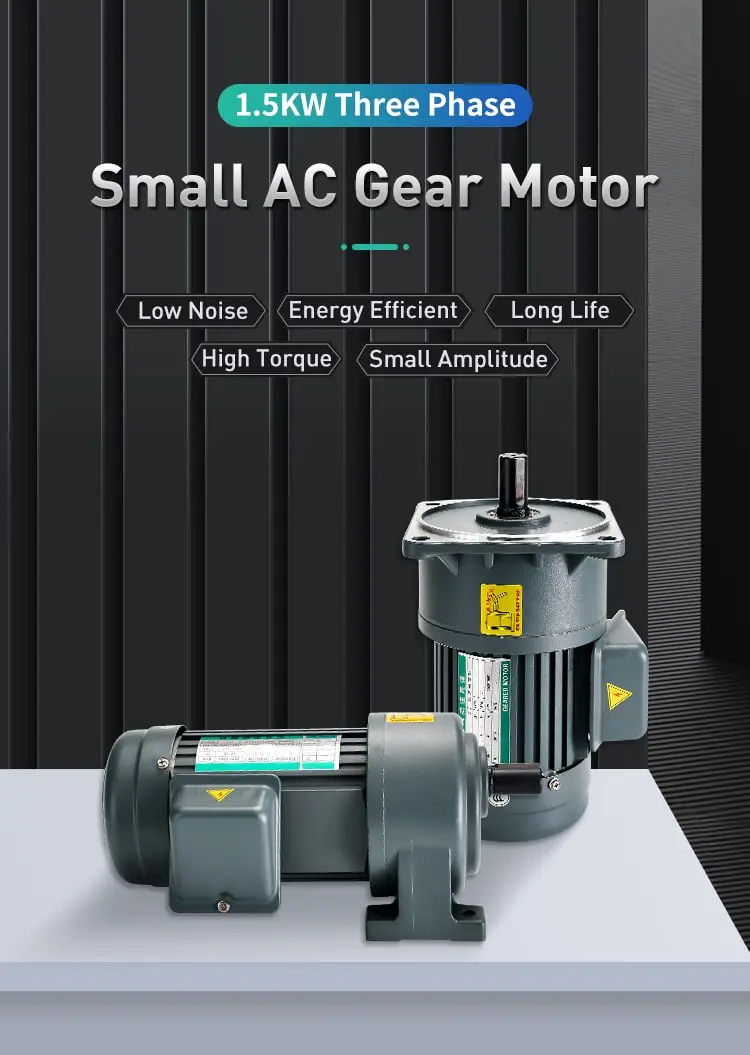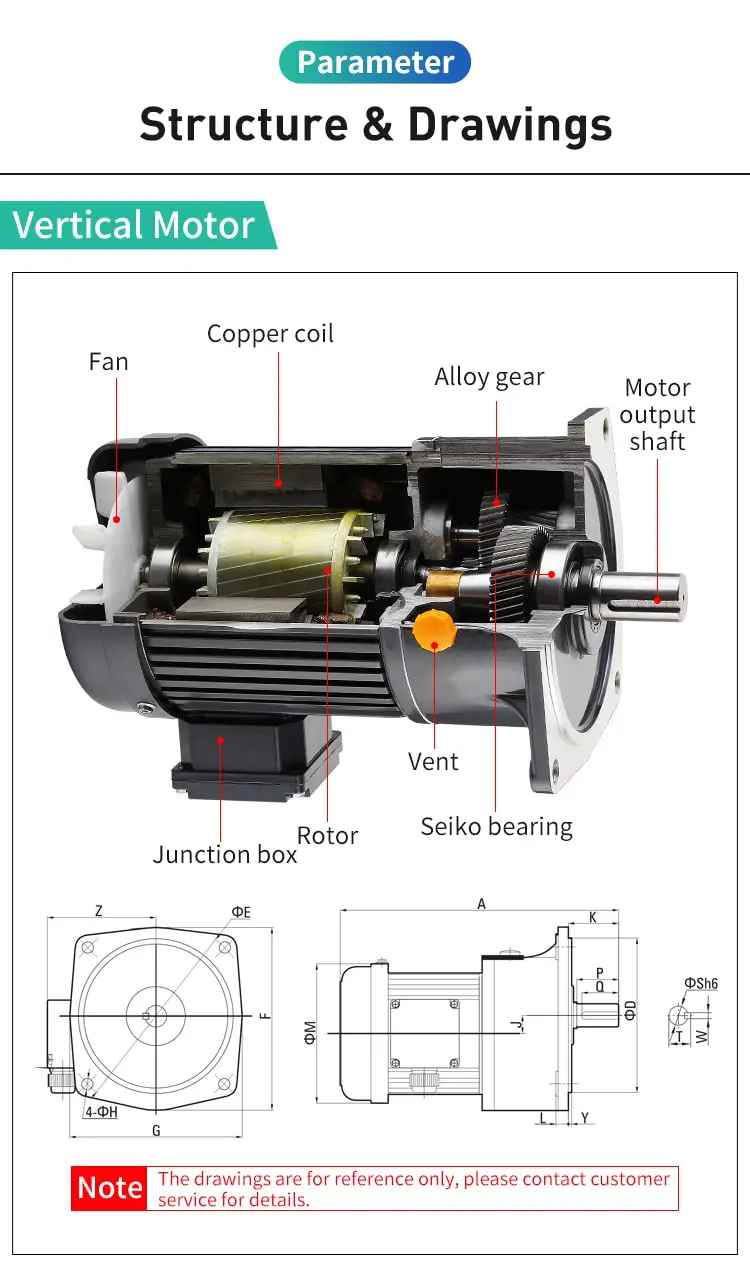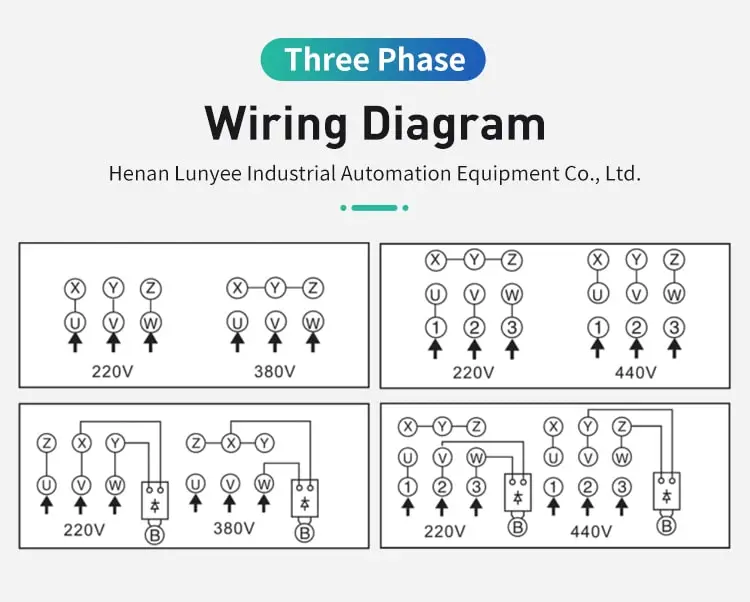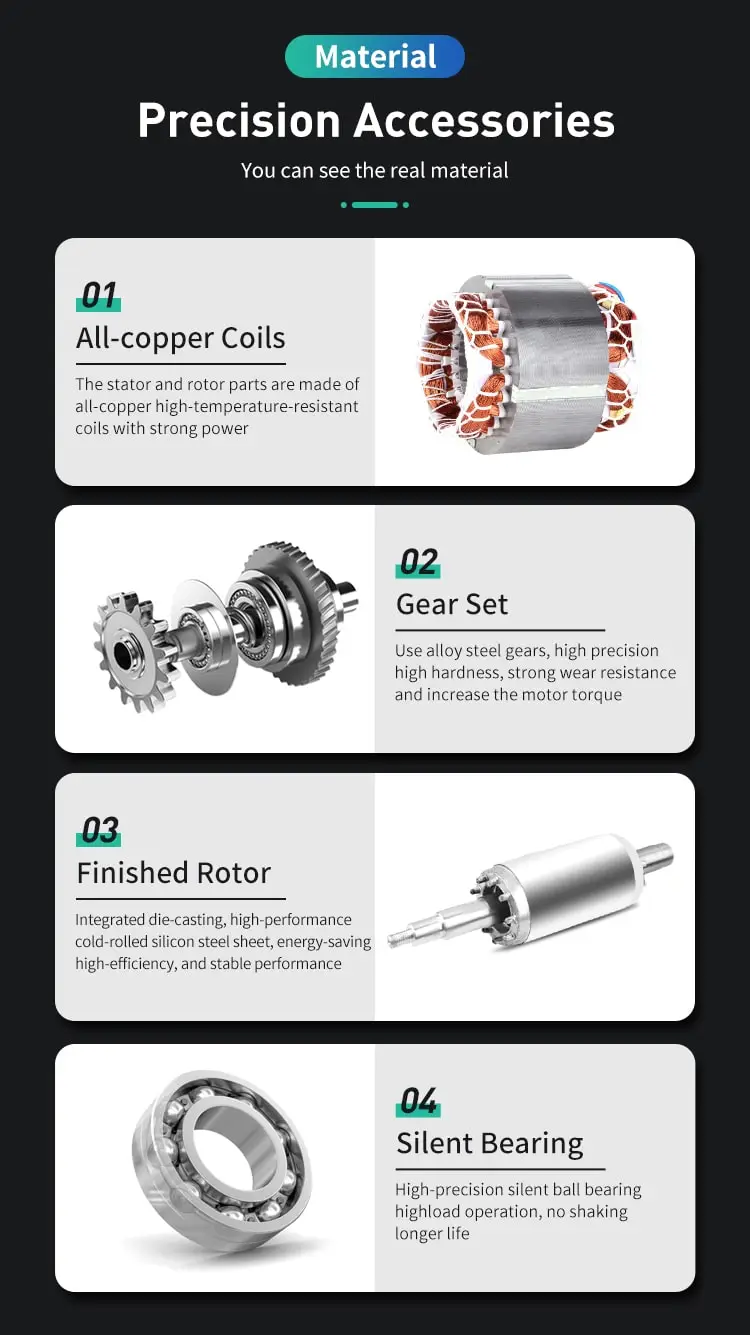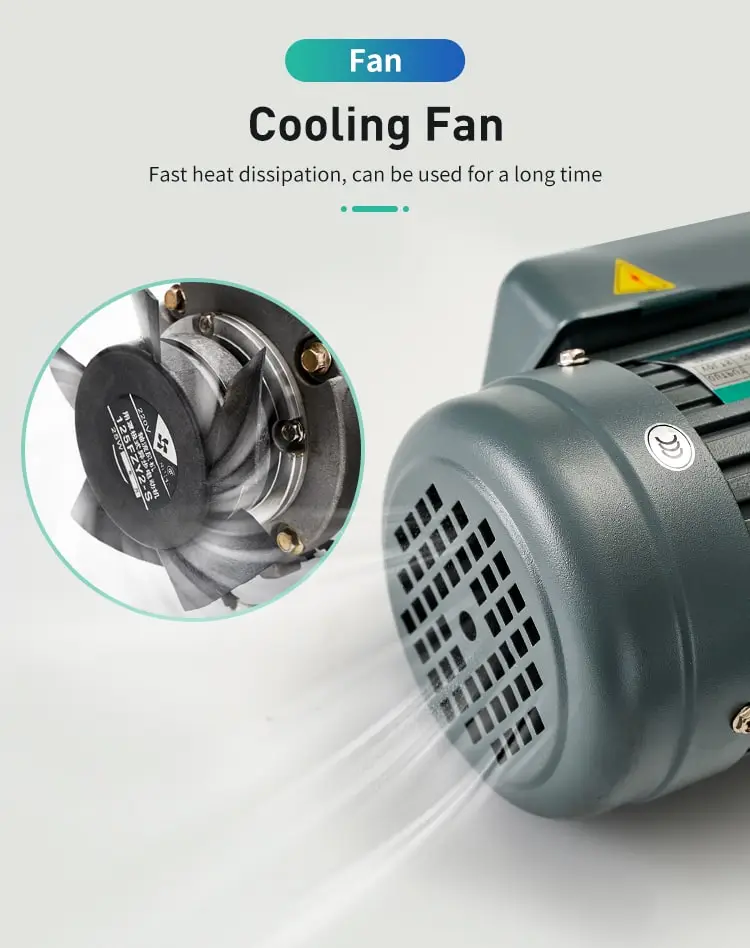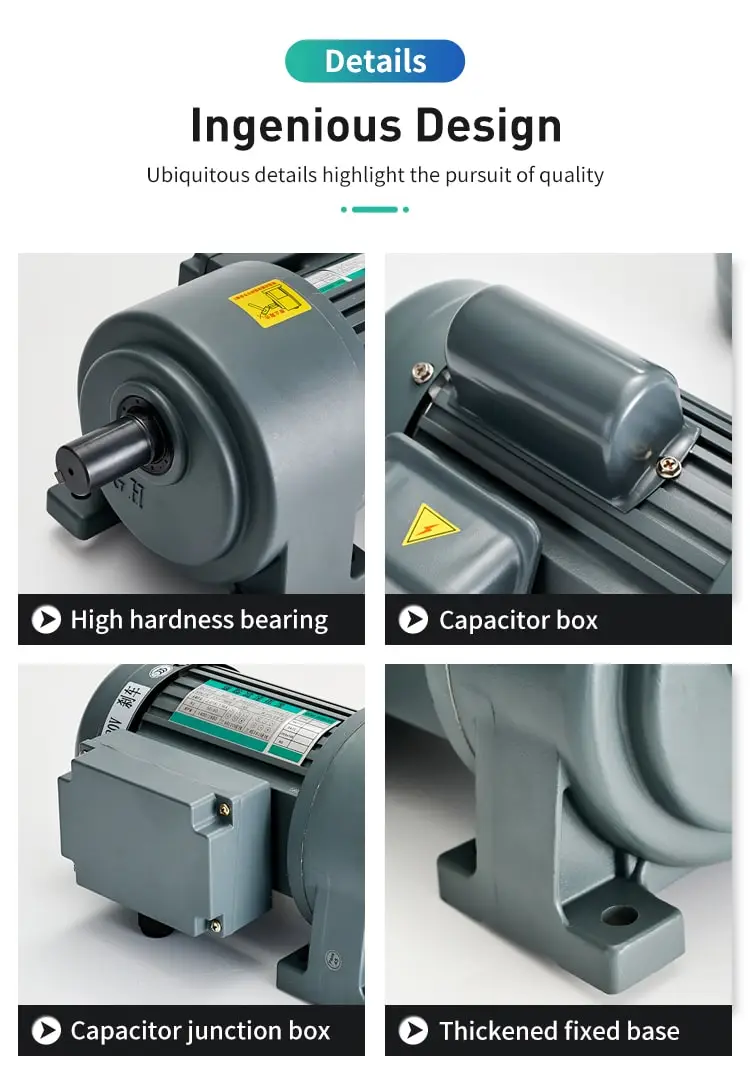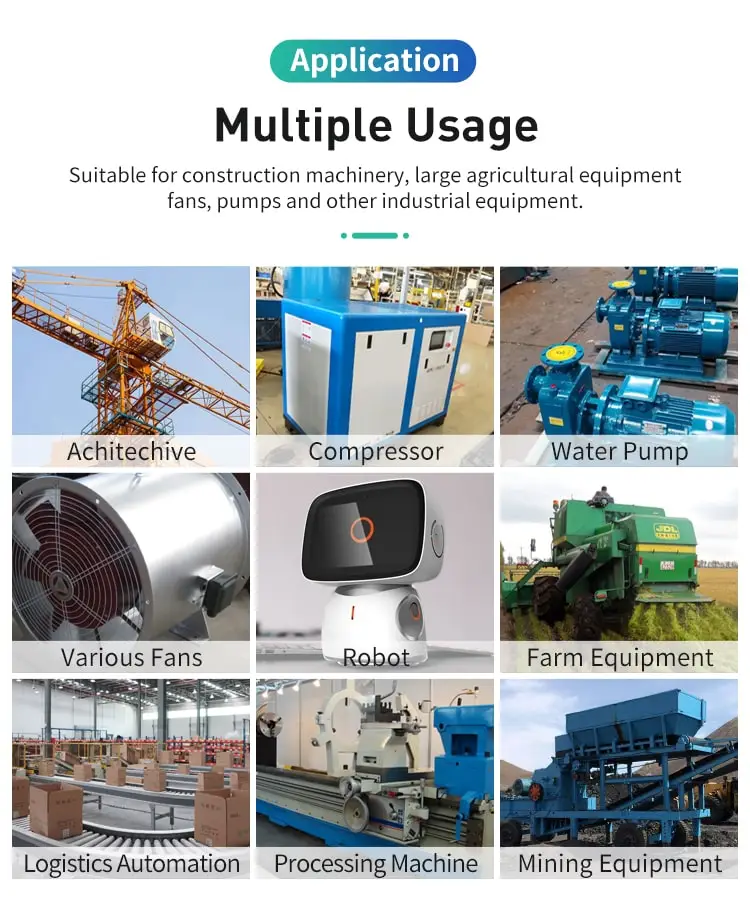By Jasmine 2023-05-12 15:22:21

#### What determines the speed of ac induction motors?

The speed of an AC induction motor is determined by the frequency of the power supply and the number of poles in the motor.

The speed of an AC induction motor is determined by a number of factors, including the frequency of the electrical power supply, the number of poles in the motor, and the load on the motor. In this article, we will explore these factors and explain how they affect the speed of an AC induction motor.

The frequency of the electrical power supply is one of the main factors that determines the speed of an AC induction motor. The frequency of the electrical power supply is typically measured in hertz (Hz) and is typically either 50 Hz or 60 Hz. A higher frequency electrical power supply will result in a higher speed for the motor, while a lower frequency electrical power supply will result in a lower speed.

The number of poles in the motor is another factor that determines the speed of an AC induction motor. The pole number of a motor refers to the number of magnetic poles it has, which in turn determines its speed and power output. A motor with a higher number of poles will have a lower speed and a higher power output, while a motor with a lower number of poles will have a higher speed and a lower power output.

The load on the motor is another factor that can affect the speed of an AC induction motor. The load on the motor refers to the amount of mechanical work that the motor is required to perform, and it is typically measured in terms of torque and horsepower. A motor with a higher load will have a lower speed, while a motor with a lower load will have a higher speed.

In conclusion, the speed of an AC induction motor is determined by a combination of factors, including the frequency of the electrical power supply, the number of poles in the motor, and the load on the motor. By understanding how these factors affect the speed of an AC induction motor, you can better select the right motor for your application and ensure optimal performance and efficiency.